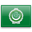Arabic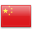Chinese (Simplified)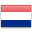Dutch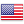English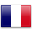French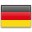German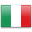ItalianPortuguese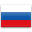Russian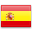Spanish
0

# Factoring semi-primes with (quantum) SAT-solvers | #itsecurity | #infosec | #hacking | #aihpState of the art classical factoring algorithms have super-polynomial expected runtime $$L_N[1/3, (64/9)^{1/3}]$$29, whereas Shor’s algorithm30 runs in expected polynomial time. This algorithm requires a fault-tolerant quantum computer and no scalable version has been implemented yet. Shor’s algorithm has profound practical implications for currently deployed public-key cryptography such as RSA and the timing of the factoring of 1024-bit, 2048-bit or even larger semi-primes is of great practical significance for both contemporary and future security systems31. Mitigations for future systems and current systems requiring long-term security are being researched by the field of post-quantum cryptography32,33,34.

An interesting notion of quantum computing has been proposed by Farhi et al.35 in the form of adiabatic quantum computers. It was suggested that adiabatic quantum algorithms may be able to outperform classical computers on hard instances of NP-complete problems36. Since then, adiabatic quantum computation (a generalization of the adiabatic optimization explored deeply by Farhi et al.) has been proven to be polynomially equivalent to quantum computation in the standard gate model37. While the possibility of super-polynomial (or even just super-quadratic) quantum speed-up for NP-hard problems remains an open question it is generally believed that quantum computers (including adiabatic quantum computers) are not able to efficiently solve NP-hard problems such as SAT. It is known that any such speed-up must go beyond pure “black-box” search38 as attempted by Farhi et al.36 and must somehow exploit additional knowledge or structure39. Note that this assumption is implicit, e.g. in the fact that post-quantum cryptographers are working on the assumption that symmetric algorithms like AES and SHA that offers n bits of security against classical attacks offer n/2 bits of security against the best known quantum attacks33 (excluding some specific attacks in the “quantum superposition” attack model40). In this section we consider the speedup that can be achieved by reducing the problem of factoring a semi-prime to an instance of an NP-hard problem which is then solved with a quantum computer.

### Faster SAT solvers

One might hope that we can apply a quantum strategy that can improve on the best known classical methods. We chose SAT solvers to represent the best classical methods as their implementations are the highly optimized result of years of research. Generic quantum searching methods can achieve at most a quadratic speed-up, and we are aware of no convincing evidence that more than a quadratic speed-up can be achieved by quantum SAT-solving methods. For example, many modern SAT solvers rely on machine-learning techniques24 and many quantum methods with a quadratic speedup are known for a variety of machine-learning algorithms41. See also42 for why the exponential speedup promised in some quantum machine-learning literature is unlikely to be achieved in real-world implementations.

A quick calculation shows that even with a quadratic speedup, this strategy is not a very efficient one. We set an upper bound on the number of operations required for the classical solver based on our results. Accounting for any internal parallelism in the processor (four arithmetic ports per processor) and assuming that the CPU was fully occupied at every clock cycle this means that $$10^{10}$$ operations were being executed every second during the solving time.

Under this assumption the expected number of operations required for classical SAT solving becomes $${2^{16.8}\times 2^{0.495n}}$$. With a quantum computer we might hope to reduce this to $$\sqrt{2^{16.8}\times 2^{0.495n}} = {2^{8.41}\times 2^{0.247n}}$$ operations. To put this in perspective, consider a quantum computer that can execute $$10^{40}$$ quantum operations per second. Note that even a classical computer with such speeds could break AES-128, SHA-256, RSA-2048 and ECC P-256 in an instant, so this is a very generous upper bound. Under these assumptions it would still take approximately a hundred times the lifetime of the universe to factor the RSA-250 number using the quantum SAT solving approach, whereas this number has been factored classically on a real machine in roughly 2700 core-years using number-theoretical methods. A visual comparison of these results are given in Fig. 6.

Note that all estimates so far are biased towards more (classical) operations per second. The reason is that we want to compute an upper bound on the speedup that can be achieved by applying Grover’s algorithm (or some alternative quadratic speedup) in order to factor numbers with SAT solving. It is almost certain that the processor executed less operations and it is very unlikely that the quadratic speedup can be applied to the full computation without any overhead of executing the algorithm. Therefore, classical algorithms will likely require less operations than reported and quantum algorithms will likely require more operations than computed.

Note also that the known speedups for quantum solvers are applied to $$T_s$$, even though the above calculation generously assumes that $$T_p(n) = 0$$ and the speedup can be applied to the full calculation time T(n). For most classical solvers it indeed holds that $$T_p(n) \ll T_s(n)$$ as n grows large enough, but for many of the adiabatic factoring methods discussed below it holds that $$T_p(n) \gg T_s(n)$$ as n grows. This means that our calculation is a significant overestimation of the maximum speedup that can be achieved with the adiabatic factoring method.

The method used for factoring with the adiabatic algorithm first reduces factorization to finding the roots in a set of integer equations in which the unknown variables are restricted to binary values, corresponding to the input bits of the prime and carry bits of the intermediate computation. This is translated to the pseudo-Boolean optimization problem by squaring all equations (so that the roots correspond to the minimum values) and summing over all equations, resulting in a quartic polynomial. This reduction was first suggested by Burges as a method for generating unconstrained optimization problems whose complexity can be easily controlled43. The adiabatic algorithm35 is particularly well suited for encoding optimization problems of this kind: the resulting sum describes a Hamiltonian whose ground state encodes the solution and every variable corresponds to a single qubit. In general it is not easy to physically initialize the system in the ground state of the problem Hamiltonian. Instead the adiabatic method intializes the system in the ground state of an easier Hamiltonian. The adiabatic theorem tells us that if we evolve the physical system from the initial Hamiltonian to the final Hamiltonian slow enough, the system will remain in its ground state. Measuring the final state will then provide the answer to the optimization problem.

To assess the power of the adiabatic algorithm it is therefore important to quantify how fast this evolution can be done. A coarse lower bound is given by the spectral width of the time-dependent Hamiltonian, but sharper bounds on the runtime so far elude us39. This has led some researchers to study the applicability of the adiabatic algorithm to some NP-complete problems36. Most evidence for a speed-up is based on noise-free simulations on small instances (for which the asymptotic behaviour might not be visible) which are chosen randomly, shedding light on typical performance for small instances. Cryptographic problems require average-case hardness in order to be practical, which is why they are so suitable for testing worst-case behaviour of algorithms that solve them, especially when the reduction to an NP-hard problem is as simple as reducing factoring to SAT as demonstrated in the previous section.

Pseudo-Boolean optimization is known to be NP-hard, meaning amongst other things that a polynomial reduction exists from the SAT problem44. Real-world demonstrations of the adiabatic algorithm suffer from additional limitations (besides noise-resistance) in the number of available qubits and multi-qubit interactions. The latter limitation means that quartic terms in the objective function cannot always be realized. Using quadratization45 each objective function can be simplified to a quadratic polynomial at the price of additional variables, giving an instance of the well-studied quadratic unconstrained binary optimization (QUBO) problem. This simplification runs in polynomial time and results in only polynomially many variables overhead, so the problems are equivalent.

However for many real-world systems the extra variables (qubits) are not available, so additional simplifications are required. This is fine as long as these simplification steps do not dominate the overall runtime of the program. More precisely we can execute polynomially many simplification operations and $$T_p(n)$$ will remain polynomial in n, thereby not significantly increasing the runtime T(n) which is dominated by the super-polynomial runtime $$T_s(n)$$. When the simplification process is allowed to have an exponential runtime it can absorb the hardness of the problem, leaving a weaker problem to be solved (trivially) in polynomial time.

#### Implementations

The first adiabatic factorization46 was implemented in 2008 using nuclear magnetic resonance (NMR) to factor 21 using three qubits. The authors fit a quadratic curve against a theoretical approximation in a noiseless model, they measure the runtime as a function of the number of input qubits (not the size of the factored number) and they only consider the small domain of seven to sixteen input qubits. The same method has factored 551 by applying some preprocessing first47. It is doubtful that such small instances are a good indicator of polynomial asymptotic behaviour.

Later work48 translates the problem of factoring 143 into a pseudo-binary optimization instance, which is an NP-hard problem44. The authors manage this by introducing the additional assumption that both factors must be of equal bitlength with the most significant bit set to one. Combining these assumption with some simplifications in the pseudo-Boolean equations simplifies the problem so that it only concerns four input bits of the prime factors. Although the used simplifications are efficient, only an upper bound of their effectiveness is given.

Subsequent research49 observes that a minor generalization of the previous method reduces the problem to four input qubits whenever the two primes composing the semi-prime differ only in two positions, which likely occurs for infinitely many semi-primes50. This provides some evidence that the simplifications do not generalize and the factored number 143 was identified as a particularly easy number to factor. In other words, this example was hand-picked from an exponentially unlikely family of semi-primes that are by design easy to factor. The authors report the number 56,153 as being the largest semi-prime factored quantumly and at the same time argue that the work has factored an arbitrarily large set of semi-primes (since they can be pre-processed into solving the same pseudo-Boolean equations). The reason for not reporting a bigger number appears to be the large runtime $$T_p$$ of the simplification process.

Much subsequent research in the adiabatic factoring field has focussed on methods such as deduc–reduc51, split-reduc52 and energy landscape manipulation53, all of which can be seen as improvements on the preprocessor runtime $$T_p$$ and none of which do any improvements on $$T_s$$.

The problems with viewing these works as relevant quantum integer factorization benchmarks is highlighted even further in the more recent paper that claims to have factored 291,311 with adiabatic quantum computation54. The authors take the above approach and reduce the problem of factoring 291,311 to the integer equations $$q_1 + q_2 – 2 q_1 q_2 = 1$$, $$q_2 + q_5 – 2 q_2 q_5 = 0$$, and $$q_1 + q_5 – 2 q_1 q_5 = 1$$. where the variables $$q_i$$ must take on binary values and represent unknown bits in the binary representation of factor $$q = 1000 q_5 01 q_2 q_1 1$$. The authors stop their simplification process at this point and fail to notice that the above equations can be further simplified to $$q_1 = 1 – q_2 = 1 – q_5$$. Both solutions $$q_1 = 0$$ and $$q_1 = 1$$ correspond with respective factors $$q = 557$$ and $$q = 523$$. In other words, the number was already factored by the simplification process and the adiabatic quantum computation was a complicated way of flipping a coin and deciding between the two factors. The above criticism of these claims to meaningful quantum factoring benchmarks was in fact already made in 201355.

A method called Variational Quantum Factoring (VQF)56 employs the same strategy for factoring, which is to reduce it to an NP-hard optimization problem. The authors are careful to ensure that preprocessing takes only polynomial time. Although the authors claim that “VQF could be competitive with Shor’s algorithm even in the regime of fault-tolerant quantum computation”, we find no convincing argument to support this conjecture. In particular, they do not provide convincing evidence that the solving step is efficient: no semi-prime larger than $$2^{15}$$ is considered by their work and they observe that “the mere presence of carry bits negatively affects the algorithm”.

The criticism from55 applies equally well against “compiled versions” of Shor’s algorithm (where partial knowledge of the factors is used to specify the quantum circuit): both implementations require much precomputation and therefore do not scale to factoring larger numbers. The problem is that both precomputations require prior knowledge of the solution. “Compiled versions” of Shor’s algorithm were never intended to scale to meaningful input sizes, as is highlighted in the abstract of the work factoring 15 with NMR: “scalability is not implied by the present work. The significance of our work lies in the demonstration of experimental and theoretical techniques”57.

The important difference is that the runtime of Shor’s algorithm is well understood and provides a super-polynomial speedup in $$T_s$$ over even the best numerical methods for factoring. As fault-tolerant hardware emerges, we can simply strip away the non-scalable optimizations. On the other hand the runtime of reducing factoring to an NP-hard problem and then solving it with (quantum) solvers is not understood very well, but the evidence provided in this work points in the direction that it cannot even compete with classical numerical methods for factoring.

### D-Wave

The D-Wave systems work by a process called quantum annealing, which can be viewed as a noisy version of adiabatic quantum computing. It has been shown that $$O(n^2)$$ qubits suffice to encode factoring into a quantum annealing instance with local interactions58. The article “Boosting integer factoring performance via quantum annealing offsets”59 describes a “boost” when comparing factoring on the D-Wave machine with annealing offsets against the D-Wave machine without annealing offsets. The largest factored number has 20 bits.

All semi-primes up to 200,000 (18 bits) have been factored with help of the D-Wave 2X by heuristically mapping the optimization problem to the Chimera graph underlying the machine60. Exponential methods from computational algebraic geometry are used for preprocessing the instances without quantification of the (asymptotic or measured) runtime so that there is no indication of the efficiency of this preprocessing step. Although some statistics on the annealing process are provided for six semi-primes, not enough information is given for a meaningful assessment on the scalability of both the efficiency and effectiveness of this method.

Integer factorization has been implemented many times on the D-Wave 2000Q by similar strategies. While early experiments only factored four semi-primes61, later work62,63 has factored more numbers by reducing the required number of qubits through more preprocessing (in polylogarithmic time). Wang et al.63 claim $$O(n^2)$$ annealing runtime without any justification; this unjustified claim seems incorrect, especially when considering the observation by Peng et al.62 that the rapidly decreasing accuracy limits the scalability of the method. None of these works presents convincing evidence that quantum annealing will find factors with significant likelihood in polynomial (or even sub-exponential) time.

A similar method was developed independently by Kieu64 and Yan et al.65. Their method translates factoring into an (NP-hard) optimization problem of minimizing $$(N – pq)^2$$ (or a similar expression), by encoding that directly in the problem Hamiltonian. Besides problems in translating the work to the Boolean logic required by the D-Wave machine66, the method has an exponential cost in energy in order to be efficient in time.ArabicChinese (Simplified)DutchEnglishFrenchGermanItalianPortugueseRussianSpanish